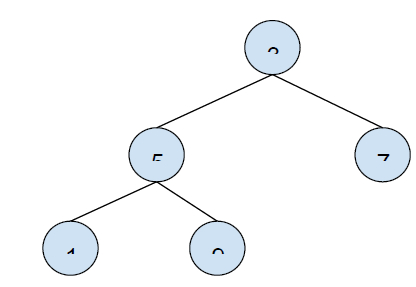# Write a program to Calculate Size of a tree - Recursion in C++

In this problem, we are given a tree and our task is to create a program to calculate the size of the tree using recursion.

The size of a tree is the total number of nodes present in the tree.

Let’s take an example to understand the problem,The size of the above tree is 5.

To find the size of the tree, we will have to add the size of left subtree and right subtree and then increment it by 1. The recursive function will be called for both left and right subtrees of the tree. And if no subtree is found return 0.

## Above example solved using this method

For finding the size of the tree,

size(3) = size(5) + size(7) + 1

size(3) = (size(1) + size(9) + 1) + 1 + 1

size(3) = (1 + 1 + 1) + 1 + 1

size(3) = 5

Program to illustrate the working of our solution,

## Example

Live Demo

#include <iostream>
using namespace std;
class node {
public:
int data;
node* left;
node* right;
};
node* insertNode(int data) {
node* Node = new node();
Node->data = data;
Node->left = NULL;
Node->right = NULL;
return(Node);
}
int findSize(node* node) {
if (node == NULL)
return 0;
else
return(findSize(node->left) + 1 + findSize(node->right));
}
int main() {
node *root = insertNode(6);
root->left = insertNode(3);
root->right = insertNode(7);
root->left->left = insertNode(1);
root->left->right = insertNode(5);
root->right->left = insertNode(2);
cout<<"The size of the given tree is "<<findSize(root);
return 0;
}

## Output

The size of the given tree is 6